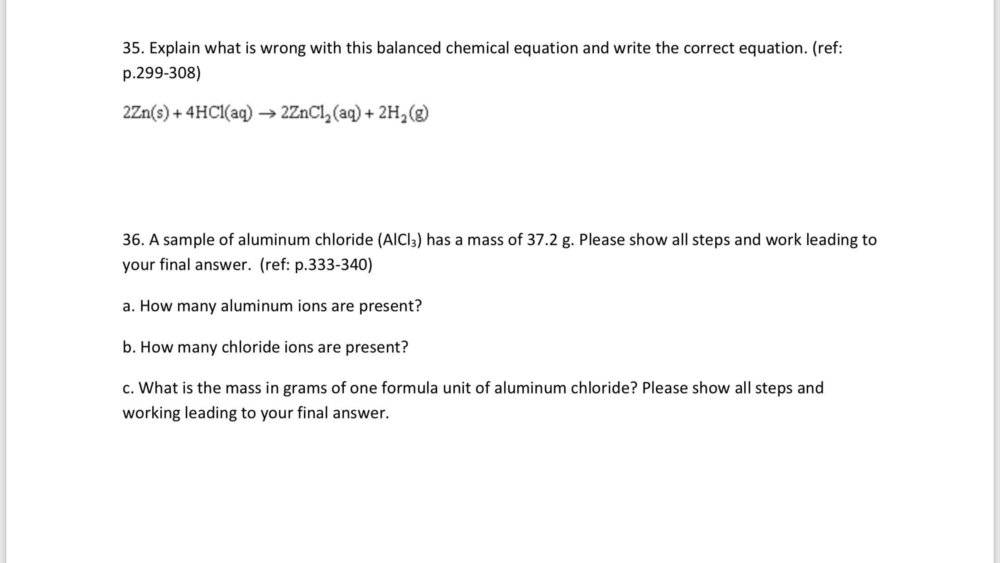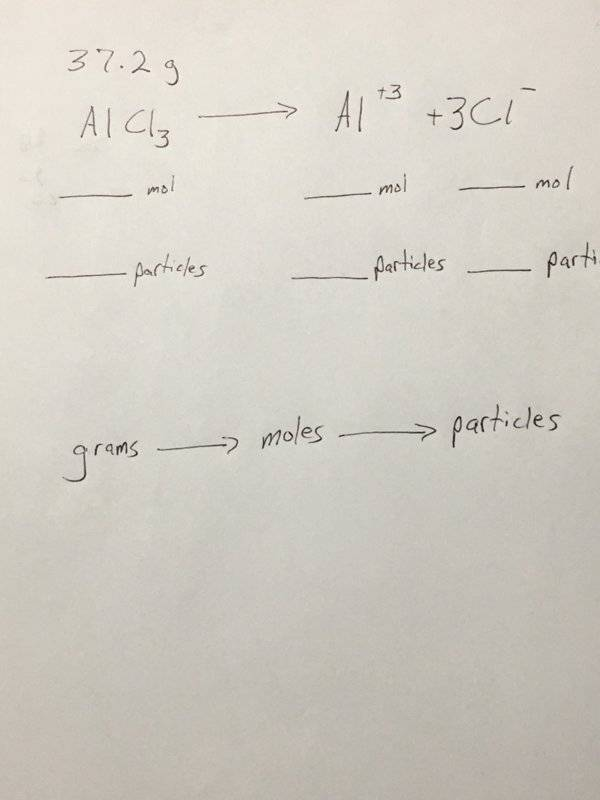# Mass to moles to particles

Homework Statement:
I need help with a question
Relevant Equations:
N/A
mass = 37.2 g AICI3 (given)
number of ions = AI3+ Ions (unknown)
number of ions = CI- Ions (unknown)
mass = g/formula unit AICI3 (unknown)
The ratio of AI3+ Ions to CI- Ions is 1:3
Molar Mass AICI3
(1 x 26.98 g/mol AI) + (3 x 35.45 g/mol CI) = 133.33 g/mol AICI3
molar mass = 133.33 g/mol AICI3
Divide the given mass of aluminum chloride by its molar mass.
37.2 AICI3/133.33 mol = 0.279 mol AICI3

Is this correct?•trurle

Homework Helper
Gold Member
So you have moles of AlCl3. Now you should write out the balanced equation showing the production of ions from the AlCl3.

Mentor
Note: it is not AICI3, it is AlCl3 (small L, not capital I). Please revise what the chemical symbols of elements are.

Homework Helper
Gold Member
You have got the number of moles of AlCl3. Which we will consider made of Al3+ and Cl- ions. The question (as I understand it) asks what is the number of these ions in the sample.

(Hint: a very large number).

You have got the number of moles of AlCl3. Which we will consider made of Al3+ and Cl- ions. The question (as I understand it) asks what is the number of these ions in the sample.

(Hint: a very large number).
1 mole of aluminum ions and 3 moles of Chloride ions

Homework Helper
Gold Member
1 mole of aluminum ions and 3 moles of Chloride ions
Nope. You really should just write out the ionization equation. It will help you visualize how to proceed.

Nope. You really should just write out the ionization equation. It will help you visualize how to proceed.
Are you looking at question 36?

Homework Helper
Gold Member
Yes.
When I was teaching AP Chemistry, I required my students to write out the relevant equation to help them visualize and organize their thoughts. I’m not being pedantic. I know it works. Try it yourself if you don’t believe me.

Last edited:
Yes.
When I was teaching AP Chemistry, I required my students to write out the relevant equation to help them visualize and organize their thoughts. I’m not being pedantic. I know it works. Try it yourself if you don’t believe me.
I don’t understand what I’m looking for.

Homework Helper
Gold Member
AB2 —-> A+2 + 2B -

This would be an example. Put what you are given above the equation and what you need to calculate beneath the equation.

Like this:

3.2 g
AB2 —-> A+2 + 2B -
? mol ?mol ?mol
? # ? # ? #

Last edited:
Homework Helper
Gold Member
I don’t understand what I’m looking for.

If I ask how many atoms there are in a mole of an element does that help make sense of the Question?

Homework Helper
Gold Member
If I ask how many atoms there are in a mole does that help make sense of the Question?
Nowadays they are asked for “how many particles” rather than how many atoms or molecules. It needlessly complicates things IMO but that’s the way it is.

1 mole = 6.02 x 10^23 atoms

Homework Helper
Gold Member
1 mole = 6.02 x 1023 atoms
1 mole = 6.02 x 1023 atoms
1 mole = 6.02 x 1023 particles

•1 mole = 6.02 x 1023 atoms
Sorry, a mistake!

AB2 —-> A+2 + 2B -

This would be an example. Put what you are given above the equation and what you need to calculate beneath the equation.

Like this:

3.2 g
AB2 —-> A+2 + 2B -
? mol ?mol ?mol
? # ? # ? #
This is confusing me. I can’t find any examples in my book.

Homework Helper
Gold Member
Which is why I’m going through it with you here.

Which is why I’m going through it with you here.
37.2 g
AICI3 —> AI+3 + 3CI -

Last edited:
Can you please give me an example of How should it be written when I get my result?

Homework Helper
Gold MemberOK. You are asked to find the number of ions of aluminum in part a. To do that you must calculate the number of moles of Al first. You have already calculated the moles of AlCl3 so you are on your way!

•Homework Helper
Gold Member
From the equation above how many moles of Al+3 are produced from each mole of AlCl3?

From the equation above how many moles of Al+3 are produced from each mole of AlCl3?
133.34g/mol?

Homework Helper
Gold Member
133.34g/mol?
No, that’s the formula weight. You notice there is a 1:1 relationship between AlCl3 and Al+3. Each mole of AlCl3 produces a mole of Al+3. Half a mole of AlCl3 produces half a mole of Al+3. How about 0.279 moles of AlCl3?

•No, that’s the formula weight. You notice there is a 1:1 relationship between AlCl3 and Al+3. Each mole of AlCl3 produces a mole of Al+3. Half a mole of AlCl3 produces half a mole of Al+3. How about 0.279 moles of AlCl3?
Yes, I’ve solved for. That

Homework Helper
Gold Member
Ok then. How many moles of Cl- do you get from 0.279 moles of AlCl3?

Ok then. How many moles of Cl- do you get from 0.279 moles of AlCl3?
Wouldn’t it be 0.279 moles too?

Homework Helper
Gold Member
Wouldn’t it be 0.279 moles too?
Each mole of AlCl3 produces how many moles of Cl-? Refer to the equation.

Each mole of AlCl3 produces how many moles of Cl-? Refer to the equation.
3?

Homework Helper
Gold Member
Right! So how many moles from 0.279 moles of AlCl3?

Right! So how many moles from 0.279 moles of AlCl3?
I don’t. Understand how to find that?

Homework Helper
Gold Member
Half a mole of AlCl3 would produce 3 X 1/2 or 1.5 mol of Cl-.

Half a mole of AlCl3 would produce 3 X 1/2 or 1.5 mol of Cl-.
So it’s 1.5?

Homework Helper
Gold Member
Yeah, IF you had half a mole of AlCl3...
So, how many moles from 0.279 moles of AlCl3?